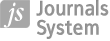Mathematics Student Teachers’ Modelling Approaches While Solving the Designed Eşme Rug Problem
,

,

,

More details
Hide details
 1 Pamukkale University, TURKEY 2 Dokuz Eylül University, TURKEY
Publication date: 2017-02-18

EURASIA J. Math., Sci Tech. Ed 2017;13(3):873–892

KEYWORDS
ABSTRACT
The purpose of the study is to analyze the mathematics student teachers’ solutions on the Eşme Rug Problem through 7-stage mathematical modelling process. This problem was designed by the researchers by considering the modelling problems’ main properties. The study was conducted with twenty one secondary mathematics student teachers. The data were collected from the participants’ written solutions related to the problem. To analyze the students’ answers through mathematical modelling process, the researchers compiled 7-stage mathematical modelling process from the literature. It was observed that the problem created an appropriate process for mathematical modelling. While examining the solutions of the participants who were informed about this 7-stage mathematical modelling process, it was generally observed that their solution approaches toward the problem decreased while progressing in modelling stages.
 eISSN: 1305-8223 ISSN: 1305-8215